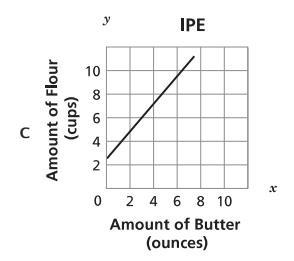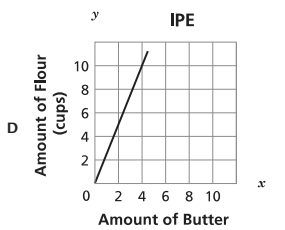Unit 3 Test Prep - Linear/Non Linear Relationships & Equations
starstarstarstarstarstarstarstarstarstar
by Maya Brown
| 13 Questions
1
1
Water flows at a constant rate out of a faucet. Suppose the volume of water that comes out in three minutes is 10.5 gallons. How many gallons of water comes out of the faucet in t minutes? Write the linear equation that represents the volume of water, V, that comes out in t minutes.
2
1
Ms. Gibson made an initial deposit of \$500 when opening a bank account. After the initial deposit, she deposited the same amount of money each month. The table below shows the total amount of money, a, she deposited into the account after a certain number of months, t, since opening it.

Which equation models the relationship between a and t ?
a = 250t
a = 500t
a = 250t + 500
a = 500t + 2503
1
Which statement explains the type of function that is represented by the equation? (Hint: Linear or Nah?)

The function is linear because it contains more than one term.
The function is linear because the variable x is raised to the second power.
The function is nonlinear because it contains more than one term.
The function is nonlinear because the variable x is raised to the second power.4
1
Which equation gives rule for the table given?
y = -3x + 57
y = x + 57
y = 3x + 57
y = -x + 57
5
1
What is the solution to the equation shown below?
x = -6
x = 4
x = -4.5
x = 9
6
1
A company performed power tests on a set of batteries of the same type. The company 10 determined that the equation y = 100 - 8.9x, where x is the number of hours of use and y is the percent of battery power remaining, models the battery life. Based on the equation, what is the best prediction of the percent of remaining power for a battery after 11 hours of use?
1.2%
2.1%
10%
97.9%
7
1
The graph of a function is shown below. Which statment is true about a section of the graph?
In Section N, the function is linear and decreasing.
In Section P, the function is linear and increasing.
In Section Q, the function is nonlinear and decreasing.
In Section R, the function is nonlinear and increasing.8
1
A cook uses 2.5 cups of flour for each ounce of butter in a recipe. Which graph, from the list below, represents the relationship between the amount of flour and the amount of butter in the recipe?
A
B
C
D9
1
Which expressions is equivilant to the one below?
2^-2
2^-1
2^6
2^7
10
1
A car traveled 36 miles in 45 minutes. The car traveled at a constant speed. If the car continues to travel at this rate, which equation can be used to determine y, the total number of miles the car will travel, in x hours?
y = 48x
y = x + 48
48y = x
48 + y = x
11
1
The points (2, -2) and (-4, 13) lie on the graph of a linear function of x. Which point also lies on the graph of this function? (Hint: Create a linear equation)
(-6, 18)
(-1, 5)
(7, 14.5)
(13, -4)
12
1
The table below shows the cost of different numbers of goldfish at a pet store. The cost is a linear function of the number of goldfish. Which statement describes the rate of change of this function?
The cost increases \$0.30 each time 1 goldfish is added.
The cost increases \$1.50 each time 1 goldfish is added.
The cost increases \$3.00 each time 5 goldfish are added.
The cost increases \$6.00 each time 5 goldfish are added.

13
1
The values given in the table below lie on the graph of a linear function. What equation represents this function?